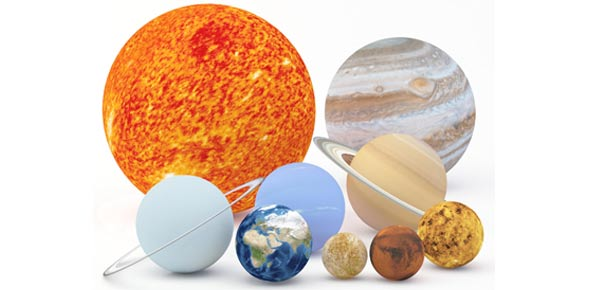# Astronomy Unit 2- Quiz 1

5 Questions | Total Attempts: 33SettingsThis quiz covers some of the basic information for unit 2.

• 1.
If the frequency of a wave is increased then what happens to the wavelength?
• A.

It increases

• B.

It decreases

• C.

It stays the same

• 2.
If the source of a wave is moving toward the observer, what is the observed change in frequency?
• A.

It increases

• B.

It decreases

• C.

It stays the same

• 3.
Which of the following electromagnetic waves have the shortest wavelength?
• A.

Visible light

• B.

• C.

Gamma Rays

• 4.
When it comes to color what color is considered the hottest?
• A.

Green

• B.

Yellow

• C.

Blue

• D.

White

• 5.
If a wave has a short wavelength, what does this tell us about the frequency?
• A.

We can't tell anything from the wavelength.

• B.

The frequency is probably low.

• C.

The frequency is probably high.

Related TopicsBack to top